## Geometric Construction

In antiquity, geometric constructions of figures and lengths were restricted to use of only a Straightedge and Compass. Although the term Ruler'' is sometimes used instead of Straightedge,'' no markings which could be used to make measurements were allowed according to the Greek prescription. Furthermore, the Compass'' could not even be used to mark off distances by setting it and then walking'' it along, so the Compass had to be considered to automatically collapse when not in the process of drawing a Circle.

Because of the prominent place Greek geometric constructions held in Euclid'sElements, these constructions are sometimes also known as Euclidean Constructions. Such constructions lay at the heart of the Geometric Problems of Antiquity of Circle Squaring, Cube Duplication, and Trisection of an Angle. The Greeks were unable to solve these problems, but it was not until hundreds of years later that the problems were proved to be actually impossible under the limitations imposed.

Simple algebraic operations such as,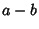,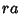(fora Rational Number),,, and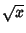can be performed using geometric constructions (Courant and Robbins 1996). Other more complicated constructions, such as the solution of Apollonius' Problem and the construction of Inverse Points can also accomplished.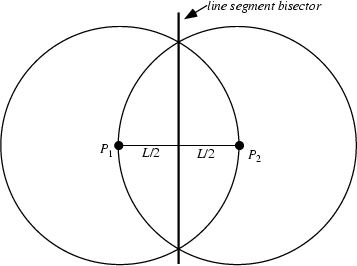One of the simplest geometric constructions is the construction of a Bisector of a Line Segment, illustrated above.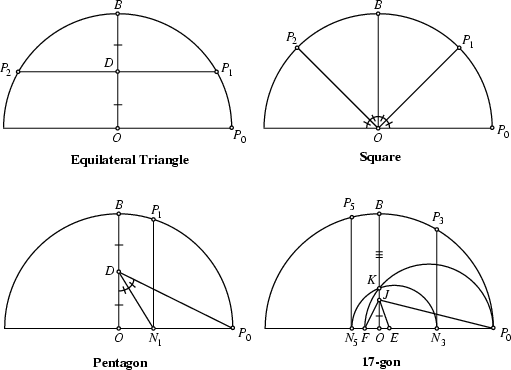The Greeks were very adept at constructing Polygons, but it took the genius of Gaußto mathematically determine which constructions were possible and which were not. As a result, Gauss determined that a series of Polygons (the smallest of which has 17 sides; the Heptadecagon) had constructions unknown to the Greeks. Gauss showed that the Constructible Polygons (several of which are illustrated above) were closely related to numbers called the Fermat Primes.

Wernick (1982) gave a list of 139 sets of three located points from which a Triangle was to be constructed. Of Wernick's original list of 139 problems, 20 had not yet been solved as of 1996 (Meyers 1996).

It is possible to construct Rational Numbers and Euclidean Numbers using a Straightedge and Compass construction. In general, the term for a number which can be constructed using a Compass and Straightedge is a Constructible Number. Some Irrational Numbers, but no Transcendental Numbers, can be constructed.

It turns out that all constructions possible with a Compass and Straightedge can be done with a Compass alone, as long as a line is considered constructed when its two endpoints are located. The reverse is also true, since Jacob Steiner showed that all constructions possible with Straightedge and Compass can be done using only a straightedge, as long as a fixed Circle and its center (or two intersecting Circles without their centers, or three nonintersecting Circles) have been drawn beforehand. Such a construction is known as a Steiner Construction.

Geometrography is a quantitative measure of the simplicity of a geometric construction. It reduces geometric constructions to five types of operations, and seeks to reduce the total number of operations (called the Simplicity'') needed to effect a geometric construction.

Dixon (1991, pp. 34-51) gives approximate constructions for some figures (the Heptagon and Nonagon) and lengths (Pi) which cannot be rigorously constructed. Ramanujan(1913-14) and Olds (1963) give geometric constructions for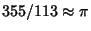. Gardner (1966, pp. 92-93) gives a geometric construction forConstructions forare approximate (but inexact) forms of Circle Squaring.

See also Circle Squaring, Compass, Constructible Number, Constructible Polygon, Cube Duplication, Elements, Fermat Prime, Geometric Problems of Antiquity, Geometrography, Mascheroni Construction, Napoleon's Problem, Neusis Construction, Plane Geometry, Polygon, Poncelet-Steiner Theorem, Rectification, Simplicity, Steiner Construction, Straightedge, Trisection

References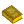Geometric Construction

Ball, W. W. R. and Coxeter, H. S. M. Mathematical Recreations and Essays, 13th ed. New York: Dover, pp. 96-97, 1987.

Conway, J. H. and Guy, R. K. The Book of Numbers. New York: Springer-Verlag, pp. 191-202, 1996.

Courant, R. and Robbins, H. Geometric Constructions. The Algebra of Number Fields.'' Ch. 3 in What is Mathematics?: An Elementary Approach to Ideas and Methods, 2nd ed. Oxford, England: Oxford University Press, pp. 117-164, 1996.

Dantzig, T. Number, The Language of Science. New York: Macmillan, p. 316, 1954.

Dixon, R. Mathographics. New York: Dover, 1991.

Eppstein, D. Geometric Models.'' http://www.ics.uci.edu/~eppstein/junkyard/model.html.

Gardner, M. The Transcendental Number Pi.'' Ch. 8 in Martin Gardner's New Mathematical Diversions from Scientific American. New York: Simon and Schuster, 1966.

Gardner, M. Mascheroni Constructions.'' Ch. 17 in Mathematical Circus: More Puzzles, Games, Paradoxes and Other Mathematical Entertainments from Scientific American. New York: Knopf, pp. 216-231, 1979.

Herterich, K. Die Konstruktion von Dreiecken. Stuttgart: Ernst Klett Verlag, 1986.

Krötenheerdt, O. Zur Theorie der Dreieckskonstruktionen.'' Wissenschaftliche Zeitschrift der Martin-Luther-Univ. Halle-Wittenberg, Math. Naturw. Reihe 15, 677-700, 1966.

Meyers, L. F. Update on William Wernick's Triangle Constructions with Three Located Points.''' Math. Mag. 69, 46-49, 1996.

Olds, C. D. Continued Fractions. New York: Random House, pp. 59-60, 1963.

Petersen, J. Methods and Theories for the Solution of Problems of Geometrical Constructions.'' Reprinted in String Figures and Other Monographs. New York: Chelsea, 1960.

Plouffe, S.. The Computation of Certain Numbers Using a Ruler and Compass.'' Dec. 12, 1997. http://www.research.att.com/~njas/sequences/JIS/compass.html.

Posamentier, A. S. and Wernick, W. Advanced Geometric Constructions. Palo Alto, CA: Dale Seymour Pub., 1988.

Ramanujan, S. Modular Equations and Approximations to.'' Quart. J. Pure. Appl. Math. 45, 350-372, 1913-1914.

Wernick, W. `Triangle Constructions with Three Located Points.'' Math. Mag. 55, 227-230, 1982.